# Class IX CBSE Mathematics Guide | Chapter 6 Lines and Angles | NCERT Solutions for Maths Exercise 6.1

CBSE Class 9 NCERT Mathematics Solutions
Lines and Angles
NCERT Mathematics Textbook Exercise 6.1 Solved
(Page 96, 97)
Q1: In the given figure, lines AB and CD intersect at O. If AOC + BOE = 70O and BOD = 40O find BOE and reflex COE.
Solution:
Given that / BOD = 40o, / AOC + / BOE = 70o
To find / BOE and  / COE.
/ BOD = / COA = 40o [vertically opposite angles]
As,  / AOC + / BOE = 70o
Or, 40o + / BOE = 70o
Or, / BOE = 30o
Also, / AOC + / COE + / EOB = 180o [angle on same straight line having same vertex].
Or, 40o + / COE + 30o = 180o
Or, / COE = 110o
Or, Reflex / COE = 360o - 180o = 250o
Q2: In the given figure, lines XY and MN intersect at O. If POY = 90O and a:b = 2 : 3, find c
Answer:  Let us assume a = 2x and b = 3x
Then, / POX = 90O [/ POY + / POX = 180O, and / POY = 90O given]
Or, a + b = 90O
Or, 2x + 3x = 90O
Or, x = 18O
a = 2x = 36O and,
b = 3x = 54O
Now, b + c = 180O [linear pair]
Or, 54O + c = 180O
Or, c = 126O
Q3: In the given figure, PQR = PRQ, then prove that PQS = PRT.
Solution:
Given:  / PQR = / PRQ (/ 1 = / 2) ….. (i)
To prove: / PQS = / PRT (/ 4 = / 3)
Proof: / 1 + / 4 = 180o and / 2 + / 3 = 180o [linear pairs]
So, / 1 + / 4 = / 2 + / 3
Or, / 2 + / 4 = / 2 + / 3 [from eq. (i)]
Or, / 4 = / 3
Or, / PQS = / PRT
Hence proved.
Q4: In the given figure, if x + y = w + z then prove that AOB is a line.
Solution:
Given that, x + y = w + z
To prove: AOB is a line.
Proof: (x + y) + (w + z) = 360o [the sum of all angles round a point is equal to 360o]
Or, (x + y) + (x + y) = 360o
Or, (x + y) = 180o
But we know that if the sum of two adjacent angles is 180o then, the non-common arms of the angles form a straight line. Therefore, AOB is a line.
Q5: In the given figure, POQ is a line. Ray OR is perpendicular to line PQ. OS is another ray lying between rays OP and OR. Prove that
Answer:
It is given that RO PQ
∠ROP = ∠ROQ = 90º
Or, POS + SOR = ∠SOQ − ∠SOR
Or, 2ROS = QOS − POS
Or, ∠ROS = ½ (QOS − POS)
Q6: It is given that XYZ = 64O and XY is produced to point P. Draw a figure from the given information. If ray YQ bisects ZYP, find XYQ and reflex QYP.
Solution:
It can be observed that PX is a line. Ray YZ stands on it.
XYZ + ZYP = 180º [linear pair]
64º + ∠ZYP = 180º
∠ZYP = 180º − 64º = 116º
Given that ray YQ bisects YZP.
Hence, ∠QYP = ZYQ = ½ YZP = 58º
Reflex QYP = 360º − 58º = 302º [the sum of all the angles round the point is 360o].
Or, ∠XYQ = XYZ + ZYQ = 64º + 58º = 122º

##### 7 comments:
Write comments
1.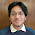2.3.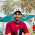helpful , thanks

4.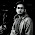cbse guid ncert solution is a nice guid i like this one very much

5.it is also very helpful!!!!!!!!

6.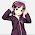I was stuck on Q5. Thanq very much ^_^ now i can do it

7.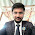It's really awesome....!!!!!